# Systems of Equations & Inequalities Chapter Exam

Exam Instructions:

Choose your answers to the questions and click 'Next' to see the next set of questions. You can skip questions if you would like and come back to them later with the yellow "Go To First Skipped Question" button. When you have completed the practice exam, a green submit button will appear. Click it to see your results. Good luck!

### Page 2

#### Question 6 6. The graphs of f(x) = 4x - 5 and g(x) = -x are shown along with their intersection point (1,-1). Based on this, what value of x will make f(x) = g(x)?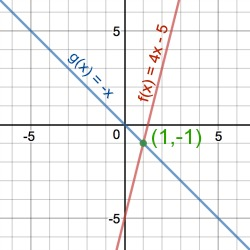#### Question 8 8. What is the solution?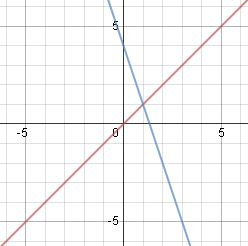#### Question 10 10. The following graph shows a system of non-linear equations with a quadratic equation and a linear equation. How many solutions does the system of equations have?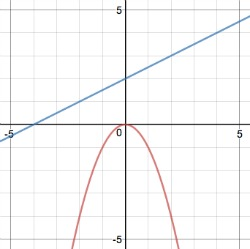### Page 3

#### Question 11 11. Consider the following non-linear system of equations, which includes a linear and a quadratic equation. What would you do first to solve the system algebraically using substitution?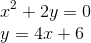#### Question 12 12. When solving the following system of equations algebraically, what would be the resulting equation? Hint: you already have the solution for y.#### Question 14 14. Which of the following is a solution to the system of equations shown in the graph below?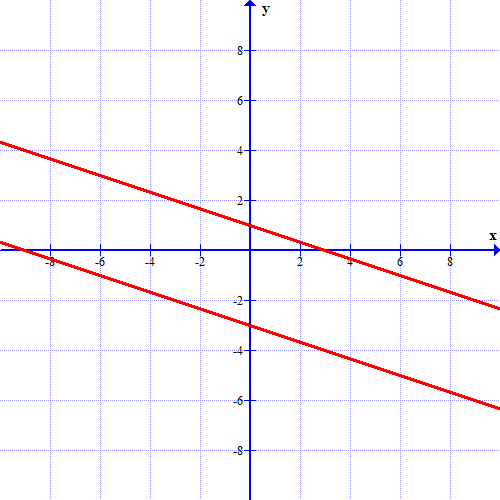### Page 6

#### Question 27 27. What is the solution?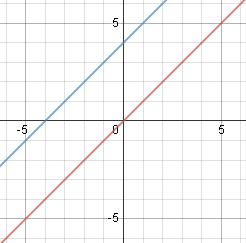#### Question 28 28. The following graph shows the graph of a system of non-linear equations with a quadratic equation and a linear equation. How many solutions does the system have?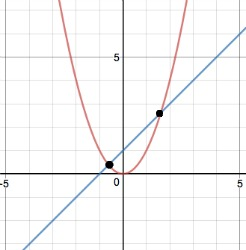#### Systems of Equations & Inequalities Chapter Exam Instructions

Choose your answers to the questions and click 'Next' to see the next set of questions. You can skip questions if you would like and come back to them later with the yellow "Go To First Skipped Question" button. When you have completed the practice exam, a green submit button will appear. Click it to see your results. Good luck!

Support